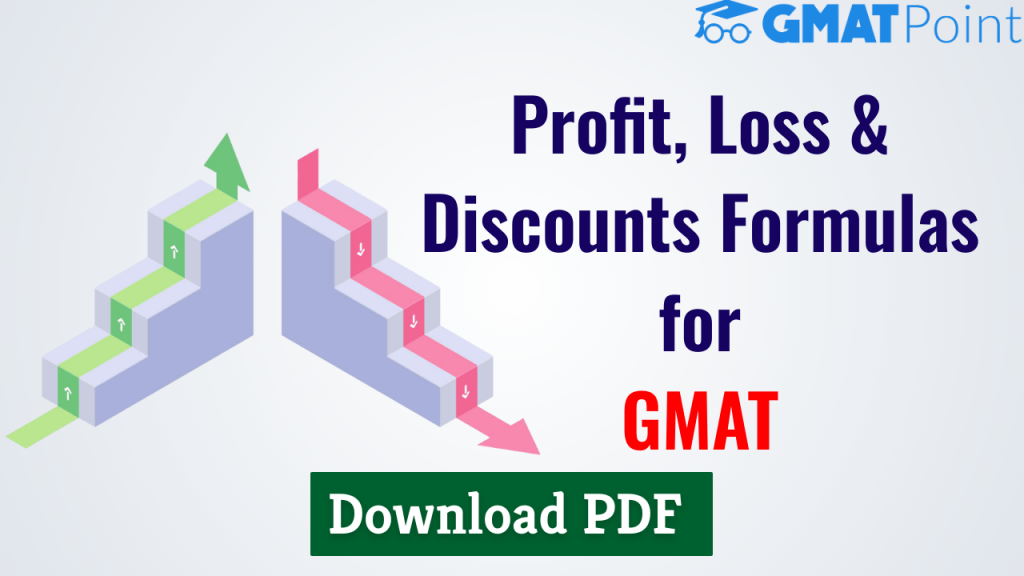# Profit, Loss & Discount Formulas for GMAT PDF# Profit, Loss & Discount Formulas for GMAT PDF

Profit, Loss, and Discount is an important topic for the GMAT, with questions asked under the Word Problem category. The number of concepts in these areas is modest, and the equations may be used to answer the majority of the problems. This document provides a variety of profit, loss, and discount formulas, recommendations, and shortcuts. We may simplify your work and save a lot of time by using the profit, loss & discount formulas. So, here are the formulas for Profit,Loss & Discount. We are providing the PDF of Profit, Loss & Discounts Formulas. You can download PDF below.

Take Free GMAT Tests

## Profit, Loss & Discount Formulas

Profit, Loss, and Discount is a prominent GMAT topic, The number of concepts in these areas is modest, and the equations may be used to answer most of the issues. This page discusses numerous profit, loss, and discount suggestions and algorithms.

Profit: The profit made when a product is sold for more than its cost price.

Loss: A loss is when a seller loses after selling a product for less than its cost price.

Cost Price (CP): A cost price is the amount paid for a product or commodity at the time of purchase. Also abbreviated as CP. This cost price is further broken down into two categories:

• Fixed Cost: The fixed cost is constant regardless of the conditions.
• Variable Cost: It may differ depending on the amount of units.

Selling Price (SP)

The Selling Price refers to the price at which a thing is sold. SP is the most common abbreviation. They are also referred to as a sale price.

Marked Price Formula (MP)

This is basically labelled by shopkeepers to offer a discount to the customers.

This Document – Profit Loss & Discount PDF covers

• Definition of Cost Price, Selling price and Marked price
• Formulas for profit percentage, Loss percentage, Percentage Discount
• Two items of same C.P sold one for some profit percent and other for same equal loss percent
• Two items of same S.P sold one for some profit percent and other for same equal loss percent
• Gain when Trader uses false weight/balance/scale
• Profit earned on Buy x get y free
•  C.P of x article is equal to the selling price of y articles

Check out the PDF for Ratio & Proportion Formulas